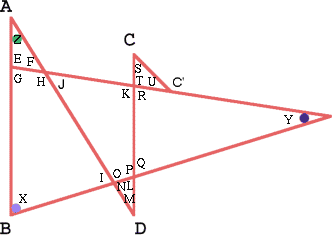#### You may also like### Calendar Capers

Choose any three by three square of dates on a calendar page...Make a set of numbers that use all the digits from 1 to 9, once and once only. Add them up. The result is divisible by 9. Add each of the digits in the new number. What is their sum? Now try some other possibilities for yourself!### Always the Same

Arrange the numbers 1 to 16 into a 4 by 4 array. Choose a number. Cross out the numbers on the same row and column. Repeat this process. Add up you four numbers. Why do they always add up to 34?

# Ratty

##### Age 11 to 14Challenge Level

If you know the sizes of the angles marked with coloured dots in this diagram which angles can you find by calculation? If in addition you know that the lines $AB$ and $CD$ are parallel which angles can you find?Congratulations on your solutions to Ian Walker, Owen Jones and Tom Embury (Y7) St James Middle School, Bury St Edmunds, to students from Y9 and Y10, The Mount School, York and to Shabbir Tejani, age 13, Jack Hunt School, Peterborough. Here is Shabbir's solution:

Angle $G = 180$ - (angle $Y$ + angle $X$)
Angle $E = 180$ - angle $G$
Angle $F = 180$ - (angle $E$ + angle $Z$)
Angle $J =$ angle $F$
Angle $H = 180$ - angle $F$
Angle $I = 360$ - (angle $X$ + angle $H$ + angle $G$)
Angle $O = 180$ - angle $I$
Angle $N = 180$ - angle $O$

These are all the angles that can be found without additional information. If we are given the added information that the lines $AB$ and $CD$ are parallel then we know:

Angle $M =$ angle $Z$
Angle $L = 180$ - (angle $M$ + angle $N$)
Angle $P = 180$ - angle $L$
Angle $K = 180$ - angle $G$
Angle $Q = 180$ - angle $P$
Angle $R = 180$ - angle $K$
Angle $T = 180$ - angle $R$

Angle S and U cannot be found.

HOWEVER if lines $CC'$ and $AD$ are parallel, then angle $M =$ angle $S$. Therefore Angle $U = 180$ - (angle $S$ + angle $T$).